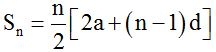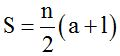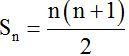All formulas and definitions from CBSE Class 10 Maths Chapter 5 – Arithmetic Progressions are available here. Check these formulas and definitions for a quick revision before the exam.

Created On: Jul 16, 2021 12:38 IST

CBSE Class 10 Maths Formulas for Chapter 5 Arithmetic Progressions

CBSE Class 10 Maths Chapter 5 all formulas and definitions are provided here. With the help of this article, students can easily revise all formulas and definitions while preparing for their exams and solve all questions efficiently to score high marks.

Check all formulas below:

1. Sequence:  It is an arrangement of numbers in a definite order.

For example:

2, 4, 6, 8,……

13, 23, 33, 43,

2. Arithmetic Progression (AP): It is a list of numbers in which each term is obtained by adding a fixed number to the preceding term except the first term.

3. Common difference (d): This fixed number is called the common difference of the AP. It can be positive, negative or zero

→ The  general form of an AP is a, a + d, a + 2d, a + 3d,…..; where a = first term of AP and  d = common difference

Also Check: CBSE Class 10 Maths Syllabus 2021-2022

4. nth Term or general term of an AP: The nth term an of the AP is given as

an = a + (n – 1) d

where

an = nth term of AP

a = first term of AP

d =  common difference

5. nth term from the last term of an AP: If l is the last term of an AP with common difference d. Then nth term is given as:

an = l – (n – 1)d

6. Sum of First n Terms of an AP:Where

a = first term,

d = common difference

n = number of terms

7. Sum of n Terms of an AP using last term:

If there are only n terms in an AP, then an = l, the last term then the sum of all n term is given as:8. Sum of First n Positive Integers (Sn = 1 + 2 + 3 + . . . + n) is given as9. Arithmetic Mean:

The Arithmetic Mean is the simple average of a given set of numbers.

→ If a, b, c are in AP, then b = (a + c)/2 is called the arithmetic mean of a and c.

→ In general, Arithmetic Mean = Sum of terms/Number of terms